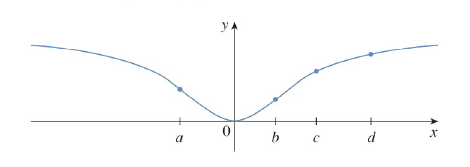Chapter 3.8, Problem 5E

Chapter
Section
Textbook Problem

# For which of the initial approximations x 1 = a , b , c , and d do you think Newton’s method will work and lead to the root of the equation f ( x ) = 0 ?To determine

For which of the initial approximation x1=a,b,c,d do you think the Newton’s method will work and lead to the root of the equation fx=0

Explanation

1) Concept:

Use newton’s method.

To find a root of y=f(x), start with an initial approximation x1 . After the first iteration of Newton’s method we will get x2, this x2 is actually the x-intercept of the tangent at that point (x1,f(x1)) and now draw a tangent at that point (x2,f(x2)) and the x-intercept at that tangent will be x3. Continue to do this till the value of xn tends to converge.

If initial approximation is given, use it for x1 to proceed.

2) Given:

Initial approximations x1=a,b,c,d

3) Calculation:

For x1=a

Draw a tangent line at a,fa

The tangent line intersect with the x-axis very close to 0

And that leads to the root of the equation fx=0

### Still sussing out bartleby?

Check out a sample textbook solution.

See a sample solution

#### The Solution to Your Study Problems

Bartleby provides explanations to thousands of textbook problems written by our experts, many with advanced degrees!

Get Started

#### Find more solutions based on key concepts